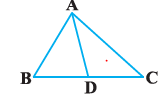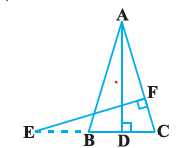# Extra questions for Similar triangles

Given below are the Similar Triangles Class 10 Extra Questions.
a. Calculation problems
b. True & False Questions
c. Multiple Choice Questions
d. Proof Questions

Question 1.
P and Q are the mid-point of the sides CA and CB respectively of a $\Delta ABC$, right angled at C. Prove that:
(i) $4AQ^2 = 4AC^2 + BC^2$
(ii)$4BP^2 = 4BC^2 + AC^2$
(iii) $4(AQ^2 + BP^2) = 5AB^2$.

Question 2.
Prove that the areas of two similar triangles are in the ratio of the squares of the corresponding altitude
Question 3.
"The areas of two similar triangles are in the ratio of the squares of the corresponding median.". Prove it
Question 4.
"The areas of two similar triangles are in the ratio of the squares of the corresponding angle bisector segments".Prove it
Question 5.
If, AD BE and CF are medians of the $\Delta ABC$, then prove that
$3 (AB^2 + BC^2 + CA^2) = 4(AD^2 + BE^2 + CF^2)$

Question 6.
In an equilateral triangle ABC, D is a point on side BC such that $BD = \frac {1}{3} BC$. Prove that $9AD^2 = 7AB^2$

Question 7.
O is a point in the interior of a triangle ABC, $OD \perp BC$, $OE \perp AC$ and $OF \perp AB$. Show that:
a. $OA^2 + OB^2 + OC^2 - OD^2 - OE^2 - OF^2= AF^2 + BD^2 + CE^2$.
b. $AF^2 + BD^2 + CE^2 = AE^2 + CD^2 + BF^2$

Question 8.
D is a point on the side BC of a triangle ABC such that $\angle ADC = \angle BAC$. Show that $CA^2 = CB.CD$Question 9.
D, E and F are respectively the mid-points of sides AB, BC and CA of $\Delta ABC$. Find the ratio of the areas of $\Delta DEF$ and $\Delta ABC$

Question 10.
E is a point on side CB produced of an isosceles triangle ABC with AB = AC. If $AD \perp BC$ and $EF \perp AC$,
prove that $\Delta ABD \sim \Delta ECF$Question 11.
O is any point inside a rectangle ABCD. Prove that $OB^2 + OD^2= OA^2 + OC^2$.

Question 12.
ABCD is a rectangle. Points M and N are on BD such that $AM \perp BD$ and $CN \perp BD$. Prove that $BM^2 + BN^2 = DM^2 + DN^2$

Question 13.
True and False
(a)All Similar triangles are congureent triangles
(b) All the equilaterals triangles are similar
(c)If the triangle ABC, AB = 5 cm, BC = 12 cm and AC = 13 cm. Then triangle a right triangle
(d) If the Two sides and the perimeter of one triangle are respectively three times the corresponding sides and the perimeter of the other triangle,Then the two triangles are similar
(e) If the Sides of two similar triangles are in the ratio 5 : 11. Areas of these triangles are in the ratio 121 : 25

Question 14.
Two triangles are similar, and the ratio of their corresponding sides is 3:5. If the length of one of the sides of the smaller triangle is 9 cm, find the length of the corresponding side of the larger triangle.
(a) 15 cm
(b) 5 cm
(c) 8 cm
(d) 11 cm

Question 15.
In a triangle ABC, the bisectors of angles A and B meet at O. If angle AOB is 120 degrees, then the angle C.
(a) 30 degrees
(b) 60 degrees
(c) 45 degrees
(d) None of these

Question 16.
Which of these is not Pythagoras triplet?
(a) 3,4,5
(b) 5,24,25
(c) 6 ,2.5 ,6.5
(d) None of these

Question 17.
The ratio of their perimeters of two similar triangles is 5:8. If the length of one of the sides of the smaller triangle is 4 cm, find the length of the corresponding side of the larger triangle.
(a) 5 cm
(b) 6.4 cm
(c) 7 cm
(d) 6.5 cm

Question 18.
In triangles ABC and DEF, $\angle B = \angle E$, $\angle F = \angle C$ and AB = 3 DE. Then, the two triangles are
(a) congruent but not similar
(b) congruent as well as similar
(c) neither congruent nor similar
(d) similar but not congruent

## Summary

This Class 10 Maths Extra questions for Similar triangles with answers is prepared keeping in mind the latest syllabus of CBSE . This has been designed in a way to improve the academic performance of the students. If you find mistakes , please do provide the feedback on the mail.Go back to Class 10 Main Page using below links

### Practice Question

Question 1 What is $1 - \sqrt {3}$ ?
A) Non terminating repeating
B) Non terminating non repeating
C) Terminating
D) None of the above
Question 2 The volume of the largest right circular cone that can be cut out from a cube of edge 4.2 cm is?
A) 19.4 cm3
B) 12 cm3
C) 78.6 cm3
D) 58.2 cm3
Question 3 The sum of the first three terms of an AP is 33. If the product of the first and the third term exceeds the second term by 29, the AP is ?
A) 2 ,21,11
B) 1,10,19
C) -1 ,8,17
D) 2 ,11,20